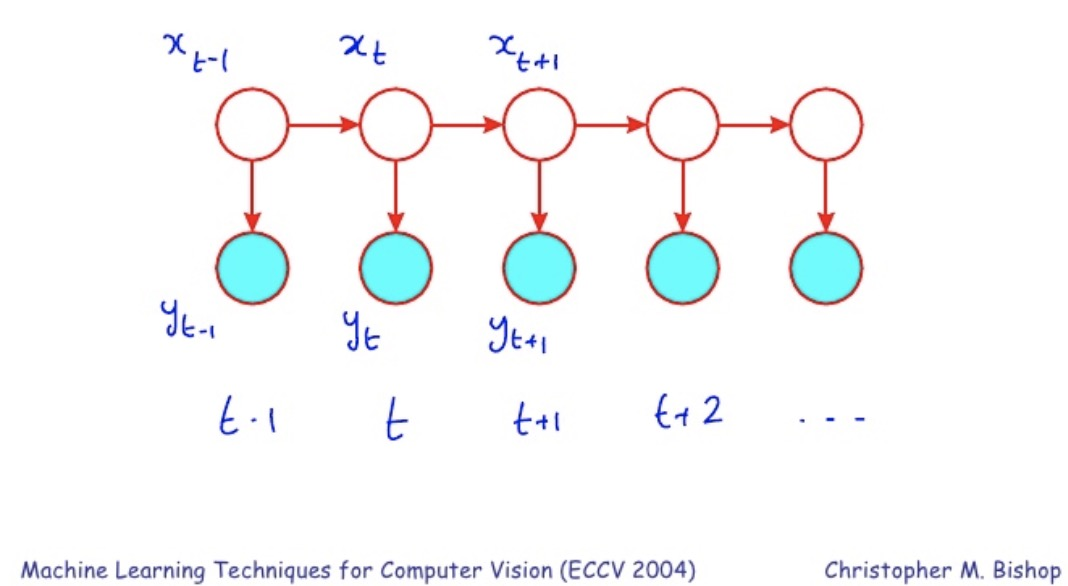Kalman Filter

Posted by GwanSiu on December 7, 2018

1. Introduction

Kalman filter and its variants, i.e., extended Kalman fiter, are one of classical algorithm and have been regarded as the optimal solution in tracking and data prediction task. Kalman filter is derived from minimum square error estimator. In this article, I will give a brief introduction to kalman filer based on the reference[1,2].

2. From HMM to Kalman Filter

Hidden markov model and kalman filter are viewed as dynamic system or state-space model. The structure of state-space model is shown as below:The assumption of state-space model is that, given latent variables, observed variables are conditional independent. In state-space model, we focus on transition probability and emission(or measurement) probability.

in state-space model, we are interested in 4 things:

1. evaluation: $p(y_{1},…,y_{t})$
2. parameter learning: $\displaystyle{\arg\max_{\theta}\log p(y_{1},…,y_{t}\vert \theta)}$
3. state decoding: $p(x_{1},…,x_{t}\vert y_{1},…,y_{t})$
4. filtering:$p(x_{t}\vert y_{1},…,y_{t})$

there are 3 kinds of dynamic model(DM) we can summary here

$p({x_{t}\vert x_{t-1}})$ $p(y_{t}\vert x_{t})$ $p(x_{1})$
Discrete state DM(HMM) $A_{x_{t-1},x_{t}}$ Any $\pi$
Linear Gaussian DM(Kalman Filter) $\mathcal{N}(Ax_{t-1}+B,Q)$ $\mathcal{N}(Hx_{t}+C,R)$ $\mathcal{N}(\mu_{0},\sigma_{0})$
non-linear,non-gaussian,DM(particle filter) $f(x_{t-1})$ $g(y_{t-1})$ $f_{0}(x_{1})$

Different from hidden markov model, in which the latent variables are discrete, in kalman fiter, latent variables are continuous, and is linear gaussian.

In this article, for kalman fiter, we focus on filtering,$p(x_{t}\vert y_{1},…,y_{t})$. The purpose of filtering is to extract information from a signal, and ignore everything else.

3. Kalman Filter(linear gaussian dynamic system)

the transition probability is

the measurement probability is

Our goal is to do the filtering $p(x_{t}\vert y_{1},…,y_{t})$. In kalman filter, it contains two stages: prediction and update.

actuall, we can expand the term $p(x_{t}\vert y_{1},…,y_{t-1})$

where the term $p(x_{t}\vert x_{t-1})p(x_{t-1}\vert y_{1},…,y_{t-1})$ is the prediction term at time $t-1$. Thus, we can see that we can adopt filtering in recursive procedure.

from above analysis, $p(x_{t}\vert y_{1},…,y_{t})$ must be gaussian distribution because $p(y_{t}\vert x_{t})$ and $p(x_{t}\vert y_{1},…,y_{t-1})$ are gaussian distribution. Thus, we need to know to $\hat{\mu_{t}}$ and $\hat{\Sigma_{t}}$. We the below idea to derive them

1. We obtain the mean and variance of $p(y_{t}\vert x_{t})$ and $p(x_{t}\vert y_{1},…,y_{t-1})$.
2. We can find the conditional distribution through their joint distribution.

Rewrite the formulation

At this time, zero-mean variable $\nabla x_{t-1}=x_{t-1}-\mathbb{E}[x_{t-1}],\nabla x_{t-1}\sim\mathcal{N}(0,\hat{\Sigma}^{-1})\Rightarrow x_{t-1}=\nabla x_{t-1}+\mathbb{E}[x_{t-1}]$.

therefore, we can see

to derive the covariance, we assume $\mathcal{COV}(x_{t-1},\omega_{t})=0,\mathcal{COV}(x_{t-1},\nu_{t})=0,\mathcal{COV}(\omega_{t},\nu_{t})=0$.

thus we can obtain that

where

we can obtain $p(x_{t},y_{t}\vert y_{1},…,y_{t-1})\rightarrow p(x_{t}\vert y_{1},…,y_{t})$ by

where $p(u)\sim\mathcal{N}(\mu_{u},\Sigma_{u})$ and $p(v)\sim\mathcal{N}(\mu_{v},\Sigma_{v})$.

from the analysis of conditonal gaussian distribution, we have:

4. Extended Kalman Filter(non-linear gaussian system)

Kalman filter: linear gaussian dynamic system

Extended kalman filter: non-linear gaussian dynamic system

we expand $F(x_{t-1})$ around a particular point $x_{t-1}^{p}$:

where $\Omega(x_{t-1}^{p})$ is higher order term, let $J_{p}=F^{\prime}(x_{t-1}^{p})$:

4.1 Transition probability-extended kalman filter

kalman filter: $x_{t}=Ax_{t-1}+\omega_{t}\quad\omega_{t}\sim\mathcal{N}(B,Q_{t})$

Mean:

Covariance

Extended kalman filter:

Mean:

Covariance:

because $x_{t-1}^{p}$ is arbitray point in time $t-1$, we can set $x_{t-1}^{p}=\hat{\mu}_{t-1}$ and simplified the formulation

Mean:

Covariance:

4.2 Measurement probability-extended kalman filter

Measurement Equation: $y_{t}=H(x_{t})+\nu_{t}\quad \nu_{t}\sim\mathcal{N}(0,R_{t})$

let $J_{p}=H^{\prime}(x_{t}^{p})$:

thus, we have

Reference

. Faragher R. Understanding the basis of the Kalman filter via a simple and intuitive derivation[J]. IEEE Signal processing magazine, 2012, 29(5): 128-132.

. Lecture-Kalman filter, Richard Xu.